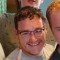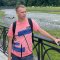# mathLast updated Apr 29th, 2019 | Page history | Improve this page | Report an issue

## FormIt math Hook¶

The math hook will allow you to have a math-based question on your form to prevent spam. It will render a math question that must be answered correctly, as follows:

12 + 23?

## Available Properties¶

Name Description Default
mathMinRange The minimum range for each number in the equation. 10
mathMaxRange The maximum range for each number in the equation. 100
mathField The name of the input field for the answer. math
mathOp1Field The name of the field/placeholder for the 1st number in the equation. op1
mathOp2Field The name of the field/placeholder for the 2nd number in the equation. op2
mathOperatorField The name of the field/placeholder for the operator in the equation. operator

## Usage¶

Include it as a hook in your FormIt call:

``````[[!FormIt? &hooks=`math`]]
``````

To make the math question required, use the call as follows:

``````[[!FormIt? &hooks=`math` &validate=`math:required`]]
``````

Paste this sample HTML in the part of the form you want to include the math question:

``````<label>[[!+fi.op1]] [[!+fi.operator]] [[!+fi.op2]]?</label>
[[!+fi.error.math]]
<input type="text" name="math" value="[[!+fi.math]]" />
<input type="hidden" name="op1" value="[[!+fi.op1]]" />
<input type="hidden" name="op2" value="[[!+fi.op2]]" />
<input type="hidden" name="operator" value="[[!+fi.operator]]" />
``````

The math question in the place of the input named "math".

NOTE: The form fields 'op1', 'op2' and 'operator' are not used anymore from FormIt version 2.2.11 and up.

### Customizing the Operator Text¶

If you don't want just "-" or "+" as the operator, and want to hide it even more from spam bots, you can use output filters to further add ambiguity to the math equation. Change the line with the equation text in it to:

``````<label>[[!+fi.op1]] [[!+fi.operator:is=`-`:then=`minus`:else=`plus`]] [[!+fi.op2]]?</label>
``````

This will render the equation like "23 plus 41?" or "50 minus 12?" instead of a -/+ symbol, making it harder for spam bots.

Support the team building MODX with a monthly donation.

The budget raised through OpenCollective is transparent, including payouts, and any contributor can apply to be paid for their work on MODX.

Backers

•••••••••••••Budget

\$217 per month—let's make that \$500!